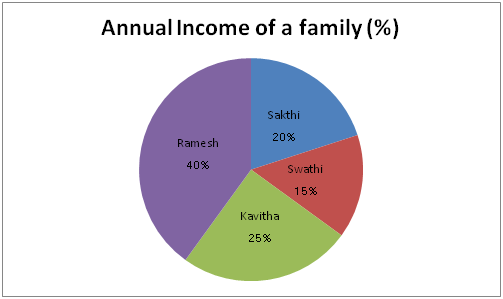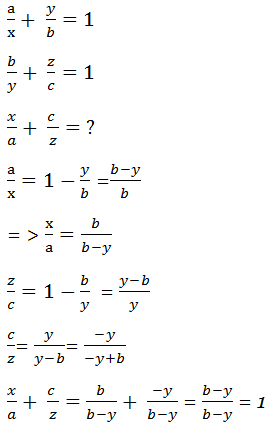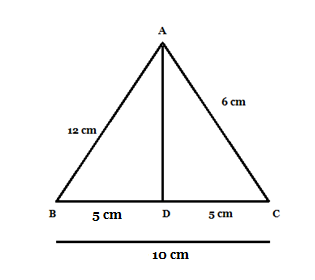# SSC CGL EXAMS 2018 | Quantitative Aptitude Practice Questions (Day-14)

Dear Aspirants, Here we have given the Important SSC Exam 2018 Practice Test Papers. Candidates those who are preparing for SSC 2018 can practice these questions to get more confidence to Crack SSC 2018 Examination.

[WpProQuiz 4602]

1) A train length 750m crosses a bridge of length 150m in 45 sec. what is the speed of the train in Kmph?

a) 72 Kmph

b) 60 kmph

c) 65 Kmph

d) &5 Kmph

2) If α, β, are the roots of the equations 2x2 – 3x + 2 = 0, form the equation whose roots are roots are α2, β2?

a) 5x2 + x – 4

b) 2x2 – x + 3

c) 5x2 – 4x + 3

d) 4x2 – x + 4

3) The four triangles by joining the pairs of midpoint of the sides of a given triangle are congruent if the given triangle is

a) A right angle triangle

b) An equilateral triangle

c) An isosceles triangle

d) None of these

4) Average of 50 numbers is 100. If the tw0 numbers 50 and 80 are expelled, then find the average of remaining numbers?

a) 99.40

b) 87.04

c) 97.40

d) 50.40

5) Somu lends Rs. 12500 to two different persons for different rate of simple interest per annum. If the difference of simple interest from the person is Rs. 25, then what is the difference between their rates of interest?

a) 0.25

b) 0.5

c) 0.75

d) 0.66

6) If a/x + y/b = 1 and b/y + z/c = 1, then value of x/a +c/z  is ?

a) 1

b) 0

c) – 2

d) – 1

7) In ABC, AB = 12 cm, BC = 6 cm, and AC =6 cm. Find the approximate length of the median from vertex A.

a) 10 cm

b) 9 cm

c) 8 cm

d) 7 cm

Direction (8 – 10): Read the instruction carefully and answer the following question.

Given pie chart illustrates the percentage of income of family members per annum. The total income of the family is 2.5 lakhs per month.8) What is the total annual income of the family, if Ramesh got 10% hike of his monthly salary?

a) 32.2 lakhs

b) 31.2 lakhs                                                                                                                  c) 35 lakhs

d) 34.1 lakhs

9) What is the difference of highest income and lowest income of the family monthly?

a) 65430

b) 65450

c) 61250

d) 62000

10) What is the ratio of swathi’s monthly income to sakthi’s monthly income?

a) 1: 2

b) 2: 3

c) 3: 4

d) 4: 5

Length of the train = 750 m

Length of the bridge = 150 m

Total distance = 750 + 150 = 900

Time = 45 sec

Speed = Distance/ Time = 900 / 45 * 18 / 5 = 60 kmph

As per given data,

α + β = -b/a = -(-3)/2 =  3/2

αβ = 2/2 = 1

For the new equations roots are α2, β2

∴ Sum of the roots α2 + β2 = (α + β)2 – 2 αβ = (3/2)2 – 2(1) = 9/4 – 2 = 1/4

Product of the roots = α2β2 = (αβ) = 12 =1

Required equation = x2 – (Sum of roots) * (products of the roots) = 0

x2 – (1/4)x +1 = 0

4x2 – x + 4 = 0

There is a property of lines joining mid-points of two sides of a triangle.
Property – Line joining the mid-points of two sides is always parallel to the third side.

If you let length of the sides to be AB, BC and AC, then

BE = CE = 1/2BC

AF = CF = 1/2AC

From the property, we can say that DE is parallel to AC. Similarly, EF is parallel to AB and DF is parallel to BC.

If you draw the figure and see, then you will notice that 3 parallelograms, AFED, FDEC and FDBE.

You can thus equate the parallel sides and we get FD = EB, EF = BD and DE = CF which are halves of the parallel sides.

Now if you check the sides of the four triangles, all of will have the same dimensions. Thus total area of ABC is sum of the areas of the 4 triangles.

Total number (x1 + x2 + x3 + x4+ ……….. x50) = 50

Average of 50 numbers = 100

Sum of Total numbers = 100 * 50 = 5000

If two numbers are expelled 50 + 80 = 130

(5000 – 130)/50 = 4870/ 50 = 97.40

S. I1 – S.I2 = ((PNR1)/ 100) – ((PNR2)/100)

S. I1 – S.I2 = PN/100 (R1 – R2)

250 = 12500 * 2/100 (R1 – R2)

R1 – R2 = 25*100/ 12500*2 = ¼ = 0.25AB =12 cm

BC = 10cm

AC = 6 cm

Median of vertex A = AD

BD = DC = ½ (BD) = ½ (10) = 5 cm

By Apollonius theorem

AB2 + AC2 = 2 (AD2 + BD2)

122 + 62 = 2 (AD2 + 52)

144 + 36 = 2(AD2 + 52)

Direction (8-10) :

Total income monthly = 2.5 lakhs

Total income annually = 2.5* 12 * (40/100) = 1 lakhs

10% hike on monthly salary = 110000

New salary of Ramesh Annually = 110000 * 12 = 13.2 lakhs

Other annual salary = 30 * (60/100) = 18 lakhs

New annual salary of the family = 13. 2 + 18 lakhs = 31.2 lakhs

Highest salary of this family = 2.5 * 12 * 40/100 = 12 lakh

Lowest salary of this family = 2.5 * 12 * 15/ 100= 4.5 lakh

12 – 4.5 = 7.5 lakh / 12 = 62,000

Sakthi’s Monthly income = 2.5 * (20/100) = 50000

Swathi’s monthly income = 2.5 * 15/100 = 37500

Ratio of swathi’s monthly ratio to sakthi’s monthly salary

= 37500 :  50000

= 3 : 4

### *******************